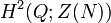# Extensions for trivial outer action of V4 on D8

This article describes all the group extensions corresponding to a particular outer action with normal subgroup dihedral group:D8 and quotient group Klein four-group.

We consider here the group extensions where the base normal subgroup$N$ is dihedral group:D8, the quotient group$Q$ is Klein four-group, and the induced outer action of the quotient group on the normal subgroup is trivial.

## Description in terms of cohomology groups

We have the induced outer action which is trivial:$Q \to \operatorname{Out}(N)$

Composing with the natural mapping$\operatorname{Out}(N) \to \operatorname{Aut}(Z(N))$, we get a trivial map:$Q \to \operatorname{Aut}(Z(N))$

Thus, the extensions for the trivial outer action of$Q$ on$N$ correspond to the elements of the second cohomology group for trivial group action:$\! H^2(Q;Z(N))$

The correspondence is as follows: an element of$H^2(Q;Z(N))$ gives an extension with base$Z(N)$ and quotient$Q$. We take the central product of this extension group with$N$, identifying the common$Z(N)$.

See second cohomology group for trivial group action of V4 on Z2, which is isomorphic to elementary abelian group:E8.

## Extensions

The table below builds on the knowledge of second cohomology group for trivial group action of V4 on Z2.

Cohomology class type Number of cohomology classes Corresponding group extension for$Q$ on$Z(N)$ Second part of GAP ID (order is 8) Corresponding group extension for$Q$ on$N$ (obtaining by taking a central product of the extension of$Q$ on$Z(N)$ by$N$, with$Z(N)$ identified) Second part of GAP ID (order is 32) Is the extension a semidirect product of$N$ by$Q$? Is the base characteristic in the semidirect product? Nilpotency class of whole group Derived length of whole group Minimum size of generating set of whole group
trivial 1 elementary abelian group:E8 5 direct product of D8 and V4 46 Yes No 2 2 4
symmetric and nontrivial 3 direct product of Z4 and Z2 2 direct product of SmallGroup(16,13) and Z2 48 Yes No 2 2 4
non-symmetric 3 dihedral group:D8 3 inner holomorph of D8 49 Yes No 2 2 4
non-symmetric 1 quaternion group 4 central product of D8 and Q8 50 No No 2 2 4
Total (--) 8 -- -- -- -- -- -- -- -- --